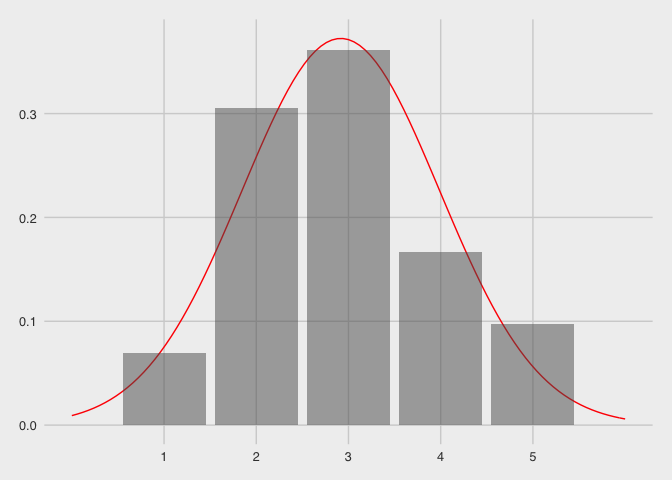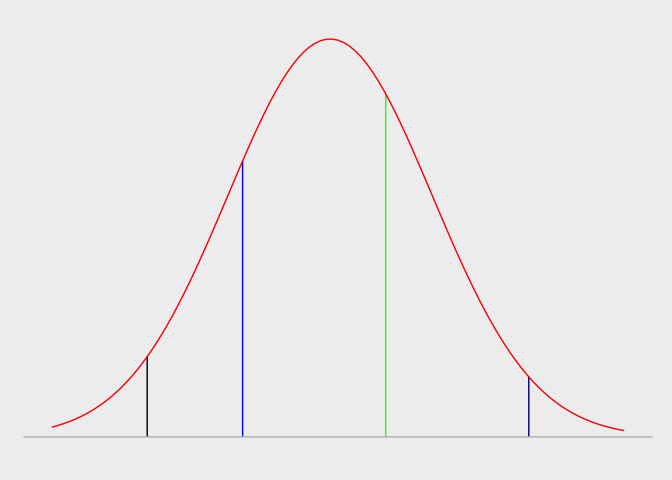Ordinal Regression in R

20 October 2016

In this note I will estimate ordinal regression model using logistic link. There are many other links possible such as probit and Weibull.

The note uses two different packages for estimating the model. The first part of the note will use ordinal package, which I recommend for your homework assignment. This is because it provides you with p-values of all the estimates in one shot. However, this package has no function to estimate marginal effects of the predictor variables.

In the second part of the note I will use MASS package to estimate the same model using the same data. Then using erer package I will get the marginal effects.

The data set we will use is incidentally another wine data! This has nothing to do with the one you are using for your homework though. This data set is available in the ordinal package.

# Install packages if you don't have them already
# install.packages(c("MASS","ordinal","erer"))
library(MASS)
library(ordinal)
library(erer)
## Loading required package: lmtest
## Loading required package: zoo
##
## Attaching package: 'zoo'
## The following objects are masked from 'package:base':
##
##     as.Date, as.Date.numeric
data(wine)

# clone the data set

w <- wine
str(w)
## 'data.frame':    72 obs. of  6 variables:
##  $response: num 36 48 47 67 77 60 83 90 17 22 ... ##$ rating  : Ord.factor w/ 5 levels "1"<"2"<"3"<"4"<..: 2 3 3 4 4 4 5 5 1 2 ...
##  $temp : Factor w/ 2 levels "cold","warm": 1 1 1 1 2 2 2 2 1 1 ... ##$ contact : Factor w/ 2 levels "no","yes": 1 1 2 2 1 1 2 2 1 1 ...
##  $bottle : Factor w/ 8 levels "1","2","3","4",..: 1 2 3 4 5 6 7 8 1 2 ... ##$ judge   : Factor w/ 9 levels "1","2","3","4",..: 1 1 1 1 1 1 1 1 2 2 ...

Our dependent variable is rating, which is an ordered factor. In case the variable that you are using is not an ordered factor, it’s preferred that you convert it into one. This is because the clm function that we will use from ordinal packages requires an ordered factor as the dependent variable.1

I will use contact and temp as the independent variables. Note that both these variables are factors and therefore R will create dummy variables for them internally. As both of them have 2 levels each (contact is either no or yes and temp is either cold or warm) , we will have one dummy variable for each independent variable. R will use the first level as the reference.

om1 <- clm(rating ~ contact + temp, data = w)
summary(om1)
## formula: rating ~ contact + temp
## data:    w
##
##  logit flexible  72   -86.49 184.98 6(0)  4.01e-12 2.7e+01
##
## Coefficients:
##            Estimate Std. Error z value Pr(>|z|)
## contactyes   1.5278     0.4766   3.205  0.00135 **
## tempwarm     2.5031     0.5287   4.735 2.19e-06 ***
## ---
## Signif. codes:  0 '***' 0.001 '**' 0.01 '*' 0.05 '.' 0.1 ' ' 1
##
## Threshold coefficients:
##     Estimate Std. Error z value
## 1|2  -1.3444     0.5171  -2.600
## 2|3   1.2508     0.4379   2.857
## 3|4   3.4669     0.5978   5.800
## 4|5   5.0064     0.7309   6.850

The model outputs the estimates for contact and temp. Internally clm function has created a fummy contactyes which will be 0 when contact = "no" and 1 when contact = "yes". Similarly tempwarm is a dummy which is 0 when temp = "cold" and 1 when temp = "warm". We notice that both contactyes and tempwarm have positive and statistically significant impact on rating of wines.

You will notice that the model also has some additional output. These are known as the threshold estimates. Next I provide some simple explanation for what these estimates are in the context of the ordered regression model.

Ordered regression model

I am going to use the “latent variable interpretation” of an ordered model. According to this interpretation, the ordinal variable is manifestation of a latent continuous variable. We don’t observe the latent variable but instead just the ordinal variable. For example, in our example, rating of the wine is an ordinal variable that might be perceived as manifestation of an underlying continuous variable of that rating. The graph below explains this concept using rating.In the above graph, the red curve is the latent variable. However, what we see is the rating which is an ordinal variable. To be sure, the latent variable doesn’t have mean of close to 3 in the above graph. I have simply superimposed the curve on the frequency chart.

Now consider the latent variable distribution below. I have indicated 4 break points. When the value of latent variable lies on the left of the black line, all we observe is 1. When the latent variable is on the left of the blue line but on the right of the black line, we observe 2. When the latent variable lies between blue and green lines, we observe 3. When the latent variable lies between the green and the dark blue line, we observe 4 and finally when the latent variable lies beyond the dark blue line we observe 5. If our characterization is right, all we have to do is to assume some distribution of the latent variable and then try to find out the 4 break points (indicated by 4 colored lines on the graph below). These break points equivalent to the thresholds that are reported by our model.I will update this note with marginal effects soon. However, you don’t need that for your homework.

1. Here is how you can make it into an ordered factor assuming your have 5 levels in your old variable. new.variable <- ordered(old.variable, levels = c(1,2,3,4,5))

Above code will create a new variable that has all the levels form the old variable. However, this will be an ordered variable with the lowest level 1 and the highest level 5. You should do this for your quality variable in the homework.